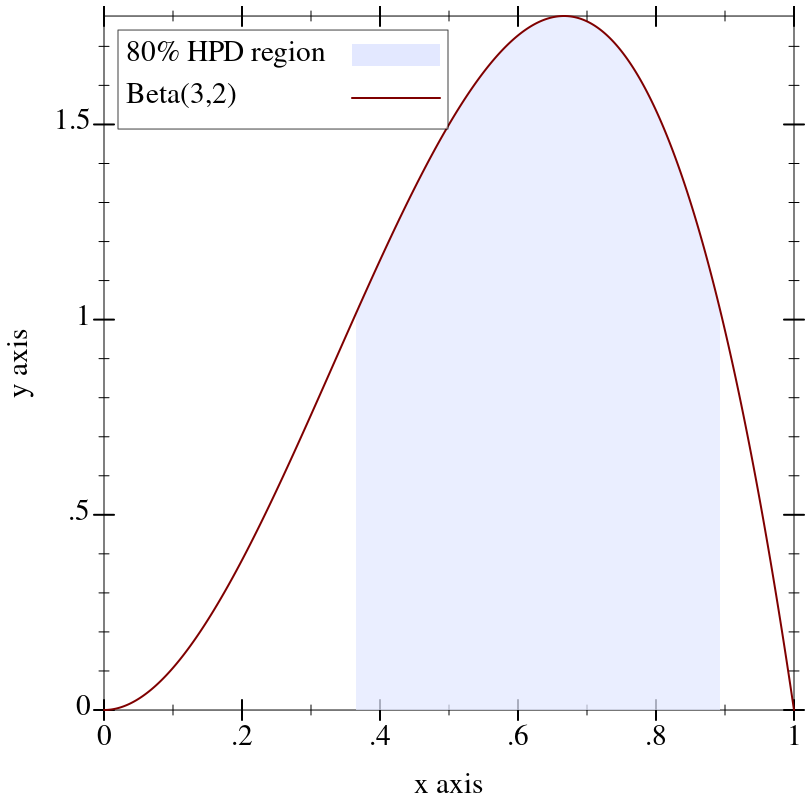#### 9.2Distribution Types and Operations

 syntax(PDF In)
The type of probability density functions, or pdfs, defined as
 (case-> (In -> Flonum) (In Any -> Flonum))
For any function of this type, the second argument should default to #f. When not #f, the function should return a log density.

 syntax(Sample Out)
The type of a distribution’s sampling procedure, defined as
 (case-> (-> Out) (Integer -> (Listof Out)))
When given a nonnegative integer n as an argument, a sampling procedure should return a length-n list of independent, random samples.

 syntax(CDF In)
The type of cumulative distribution functions, or cdfs, defined as
 (case-> (In -> Flonum) (In Any -> Flonum) (In Any Any -> Flonum))
For any function of this type, both optional arguments should default to #f, and be interpreted as specified in the description of cdf.

 syntax(Inverse-CDF Out)
The type of inverse cumulative distribution functions, or inverse cdfs, defined as
 (case-> (Real -> Out) (Real Any -> Out) (Real Any Any -> Out))
For any function of this type, both optional arguments should default to #f, and be interpreted as specified in the description of inv-cdf.

 type(distribution In Out)
 struct(struct distribution (pdf sample)) pdf : (PDF In) sample : (Sample Out)
The parent type of distribution objects. The In type parameter is the data type a distribution accepts as arguments to its pdf. The Out type parameter is the data type a distribution returns as random samples.

Examples:
 > (distribution? (discrete-dist '(a b c))) #t > (distribution? (normal-dist)) #t > ((distribution-pdf (normal-dist)) 0) 0.39894228040143265 > ((distribution-sample (normal-dist))) 0.30042678521723704

See pdf and sample for uncurried forms of distribution-pdf and distribution-sample.

Type Examples:
> (ann (poisson-dist) (distribution Real Real))
 - : #(struct:distribution ((->* (Real) (Any) Flonum) (case-> (-> Real) (-> Integer (Listof Real)))))

(poisson-dist 0.5)

 type(ordered-dist In Out)
 struct(struct ordered-dist distribution (cdf inv-cdf min max median)) cdf : (CDF In) inv-cdf : (Inverse-CDF Out) min : Out max : Out median : (Promise Out)
The parent type of ordered distribution objects.

Similarly to distribution, the In type parameter is the data type an ordered distribution accepts as arguments to its pdf, and the Out type parameter is the data type an ordered distribution returns as random samples. Additionally, its cdf accepts values of type In, and its inverse cdf returns values of type Out.

Examples:
 > (ordered-dist? (discrete-dist '(a b c))) #f > (ordered-dist? (normal-dist)) #t

The median is stored in an ordered-dist to allow interval probabilities to be computed accurately. For example, for d = (normal-dist), whose median is 0.0, (real-dist-prob d -2.0 -1.0) is computed using lower-tail probabilities, and (real-dist-prob d 1.0 2.0) is computed using upper-tail probabilities.

Type Examples:
> (ann (poisson-dist) (ordered-dist Real Real))
 - : #(struct:ordered-dist ((->* (Real) (Any) Flonum) (case-> (-> Real) (-> Integer (Listof Real))) (->* (Real) (Any Any) Flonum) (->* (Real) (Any Any) Real) Real Real (Promise Real)))

(poisson-dist 0.5)

 syntax
The parent type of real-valued distributions, such as any distribution returned by normal-dist. Equivalent to the type (ordered-dist Real Flonum).

 procedure(pdf d v [log?]) → Flonum d : (dist In Out) v : In log? : Any = #f
An uncurried form of distribution-pdf. When log? is not #f, returns a log density.

Examples:
 > (pdf (discrete-dist '(a b c) '(1 2 3)) 'a) 0.16666666666666666 > (pdf (discrete-dist '(a b c) '(1 2 3)) 'a #t) -1.791759469228055

 procedure(sample d) → Out d : (dist In Out) (sample d n) → (Listof Out) d : (dist In Out) n : Integer
An uncurried form of distribution-sample.

Examples:
 > (sample (exponential-dist)) 1.0382483313472735 > (sample (exponential-dist) 3) '(0.35131701359851364 1.0399739286006098 4.467147025654893)

 procedure(cdf d v [log? 1-p?]) → Flonum d : (ordered-dist In Out) v : In log? : Any = #f 1-p? : Any = #f
An uncurried form of ordered-dist-cdf.

When log? is #f, cdf returns a probability; otherwise, it returns a log probability.

When 1-p? is #f, cdf returns a lower-tail probability or log probability (depending on log?); otherwise, it returns an upper-tail probability or log-probability.

 procedure(inv-cdf d p [log? 1-p?]) → Out d : (ordered-dist In Out) p : Real log? : Any = #f 1-p? : Any = #f
An uncurried form of ordered-dist-inv-cdf.

When log? is #f, inv-cdf interprets p as a probability; otherwise, it interprets p as a log probability.

When 1-p? is #f, inv-cdf interprets p as a lower-tail probability or log probability (depending on log?); otherwise, it interprets p as an upper-tail probability or log probability.

 procedure(real-dist-prob d a b [log? 1-p?]) → Flonum d : Real-Dist a : Real b : Real log? : Any = #f 1-p? : Any = #f
Computes the probability of the half-open interval (a, b]. (If b < a, the two endpoints are swapped first.) The log? and 1-p? arguments determine the meaning of the return value in the same way as the corresponding arguments to cdf.

 procedure(real-dist-hpd-interval d p) → (Values Flonum Flonum) d : Real-Dist p : Real
Finds the smallest interval for which d assigns probability p, if one exists.

Examples:
> (define d (beta-dist 3 2))
> (define-values (x0 x1) (real-dist-hpd-interval d 0.8))
 > (plot (list (function-interval (λ (x) 0) (distribution-pdf d) x0 x1 #:line1-style 'transparent #:line2-style 'transparent #:label "80% HPD region") (function (distribution-pdf d) 0 1 #:label "Beta(3,2)")))